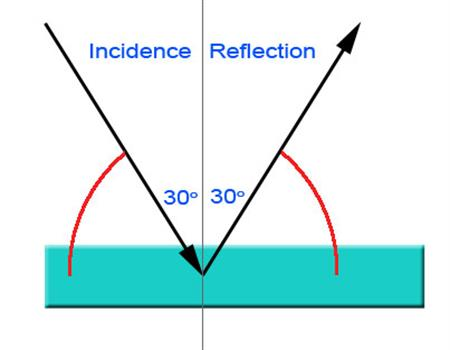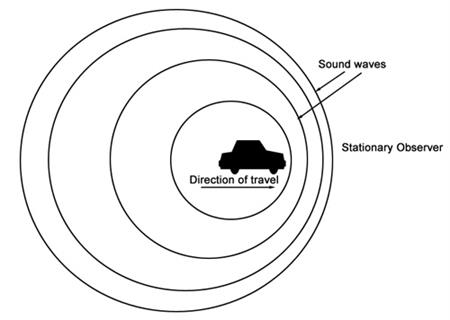# Physics

Study physics at home by distance education to understand physical sciences and study or work in physics or engineering.

Course Code BSC206 S2 100 hours Statement of Attainment

 Tweet

## It's Easy to Enrol

Select a Learning Method

I am studying from...

Enable Javascript to automatically update prices.

All prices in Australian Dollars.

Click on Enrol Now to See Our Payment Plans Available. No Obligation.

Courses can be started at any time from anywhere in the world!A knowledge of Physics is Fundamental to Most Jobs Today

If you didn't learn enough about physics while at school; this can be an opportunity to learn now.
• Start any time
• Study from anywhere and at your own pace
Develop a foundation to applying theoretical physics in any world situations, from engineering and environmental management to rural industries and health sciences.
Examining objects in motion and understanding the concepts of displacement, velocity, speed and acceleration is a great way to understand how physics work.
This course guides you through a journey of discovery, through reading, observing things, interacting with knowledgeable and experienced academics and discovering new ways of looking at the physical world around you.

## Lesson Structure

There are 10 lessons in this course:

1. Review of Basic Algebra
• Introduction
• Equations and formulae
• Variables
• Graphing
• Geometry
• Triangles
• Basic formulae
• Logarithms and exponentials
• Trigonometry
2. Introduction: Scope and Nature of Physics
• Observing, measuring, modeling, predicting
• Units of measurement
• Converting between units
• Precision of measurements and identifying significant digits
3. Forces and Mechanics
• Physics and motion
• Displacement
• Speed and velocity
• Acceleration
• Force
• Force of gravity
• Work
• Power
• Energy
4. Waves
• What are waves
• Properties of waves: longitudinal waves, transverse waves
• Wave terminology
• Relationship of frequency or period
• Wave speed
• Sound waves
• Sound spectrum
• Measuring sound
• Speed of sound
• Doppler effect
• Standing waves and resonance
5. Electricity and Magnetism
• Electrostatics
• Conductors and insulators
• How to make an electroscope
• Coulomb's law
• The electric field
• Electricity and electric circuits
• Current
• Voltage
• Resistance
• Power
• Ohm's law
• Circuits: series, parallel
• Magnets
• Magnetic forces
• Ferromagnetism
• Creating magnets
• Earth's magnetic fiels
• Geomagnetic reversal
• Electromagnetism
• Electromagnetism and solenoids
• Electric motors
• Magnetic force
• Right hand rule
• Inductors
• Lenz's law
6. Energy and Work
• What is energy
• Mechanical energy
• Potential energy
• Kinetic energy
• Conservation of total energy and mechanical energy
• Converting kinetic energy into potential energy
• Work and force
• Conservative and non conservative forces
• Conservation of mass energy
7. Fundamentals of Thermodynamics
• Temperature measurement units
• Fahrenheit
• Celsius
• Kelvin
• Converting between units
• What is heat
• Heat transfers: thermal equilibrium
• Thermal expansion and thermal contraction
8. Light and Optics
• What is light
• Reflection
• Refraction
• Demonstration of refraction
• Index of refraction
• Difraction
• The electromagnetic spectrum
• How a rainbow forms
• What are mirrors
• Flat mirrors
• Convex mirrors
• Concave mirrors
• Lenses
• Converging lenses
• Diverging lenses
• Structure of matter
• The periodic table
• Nuclear medicine -diagnostic and therapy
• Archeological and geological dating
• Half life
• Power generation
• Cancer and burns caused by radiation
10. Astronomy, Cosmology and Astrophysics
• What is astronomy
• The pioneers of astronomy
• The branches of astronomy
• Sub fields of astronomy
• Astronomy in our daily life
• The most important discoveries in astronomy
• What is Cosmology
• How did cosmology evolve
• Hubbles law
• Cosmological principle
• Calculate the age of the universe using the Hubble constant
• What is astrophysics

Each lesson culminates in an assignment which is submitted to the school, marked by the school's tutors and returned to you with any relevant suggestions, comments, and if necessary, extra reading.

## What sorts of things will you learn in this course?Maths is the language of physics, that’s why any Physics course needs to contain "some" maths.
This is not a maths course though! (We review basic and relevant maths in the first lesson).

Much of physics is about explaining physical phenomena and being able to create predictive mathematical models or theories to explain why things occur as they do and be able to predict future results in a reliable manner.

Learn to Understand Motion

Examining objects in motion and understanding the concepts of displacement, velocity, speed and acceleration is a great way to understand how physics work. It is the study of motion which began since 400 years that gave birth to the science of physics, and is often known as “kinematics”. There are only a few mathematical equations that you need to know in this section and they are relatively simple and straight forward and easy to remember.

What are Waves?

Simply put waves are a form of energy travelling from one place to another; for example think of the sun, it is very far away yet its light and heat reaches earth, think of hitting a snare drum; the sound travels to your ears. These are only two examples of waves but there are many more. Unfortunately sound waves and radio waves can’t really be seen, this makes them hard to describe, however one can visualize how waves behave when dropping a stone into water.

What are Electrostatics?

Over the past few decades, people’s dependency on electricity has witnessed an exponential growth, compared with the past century where people only used a few electric lights. However, scientists began to study and research electricity long before electric lamps were invented. These studies and observations date back to the days of ancient Greeks when they observed that rubbing amber would allow it to attract smaller objects such as feathers. To better understand electricity, we need to start with understanding electrostatics which is the study of electric charges at rest.

Understanding Energy

The energy of an object can be defined as the potential of that object to do work. This has relevance to kinetic energy, potential energy and other types of energy, such as chemical, electrical, nuclear and thermal energy. To further understand the concept of energy, consider a ball rolling on the ground. If you apply a force to the ball and increase its rolling speed, you are actually doing work on the ball which results in an increase in the ball’s energy. So as you can see, work and energy are inter-related and in this lesson you will learn how to calculate the work done on an object as well as the amount of potential and kinetic energy that object possesses at any point in time.

## WHY STUDY PHYSICS?

Physics is the foundation of so much of our daily lives. Machines, tools and equipment that we use every day, both at work, and in our private lives, are commonly designed, built and used by applying the principles of physics. Without physics, we wouldn't have computers, cameras, broadcasting (TV and radio), plumbing or electric lighting.
An understanding of physics allows construction workers to move things more easily and build things more soundly.
Physics even allows athletes to better understand how the human body moves.

This is an excellent foundation course for many industries; from engineering to photography and farming to transport.

## MAKE THE BEST DECISION

Let us help with our Free Career and Course Counselling Service.

Contact one of our science academics.
Learn from our experience

or Enrol -go to top of the page for enrolment (right column)

## Start Now!Bob James

Horticulturalist, Agriculturalist, Environmental consultant, Businessman and Professional Writer. Over 40 years in industry, Bob has held a wide variety of senior positions in both government and private enterprise. Bob has a Dip. Animal Husb, B.App.Sc.,Dr. Sherif Sakr

Research Scientist and University Lecturer in Computer Science and Engineering. Sherif has a PhD in Computer Science, MSc, BSc.Barbara Seguel

Teacher and Researcher, Marine Scientist, Tourism and Outdoor recreation guide, Health and Safety Coordinator & Production Manager for Fisheries, National Park Staff/Farmer, Laboratory technical aide, Zoo, Wildlife and Marine Park assistant. Barbara has w